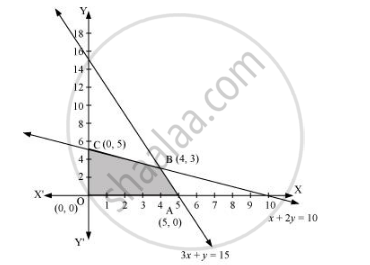Share

# Solve the Following Linear Programming Problems Graphically: Maximise Z = 3x + 2y Subject to X + 2y ≤ 10, 3x + Y ≤ 15, X, Y ≥ 0. - CBSE (Science) Class 12 - Mathematics

ConceptLinear Programming Problem and Its Mathematical Formulation

#### Question

Solve the following Linear Programming Problems graphically:

Maximise Z = 3x + 2y subject to x + 2y ≤ 10, 3x + y ≤ 15, x, y ≥ 0.

#### Solution

The feasible region determined by the constraints, x + 2y ≤ 10, 3x + ≤ 15, x ≥ 0, and y≥ 0, is as follows.The corner points of the feasible region are A (5, 0), B (4, 3), and C (0, 5).

The values of Z at these corner points are as follows.

 Corner point Z = 3x + 2y A(5, 0) 15 B(4, 3) 18 → Maximum C(0, 5) 10

Therefore, the maximum value of Z is 18 at the point (4, 3).

Is there an error in this question or solution?

#### APPEARS IN

Solution Solve the Following Linear Programming Problems Graphically: Maximise Z = 3x + 2y Subject to X + 2y ≤ 10, 3x + Y ≤ 15, X, Y ≥ 0. Concept: Linear Programming Problem and Its Mathematical Formulation.
S